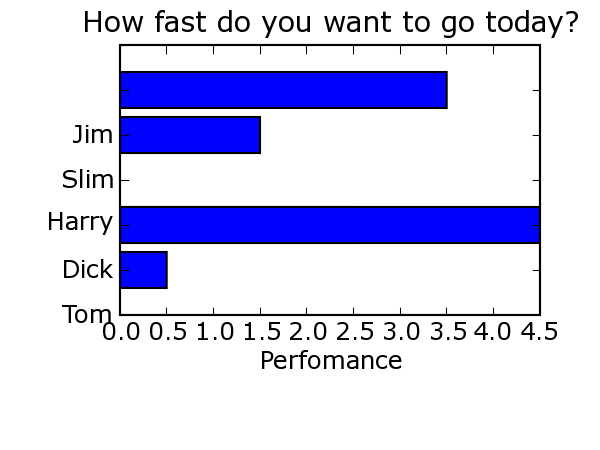# barh Chart - Not lining up

Hi guys,

I can't figure out why my code below is generating the attached image.
What am I doing wrong? I tried to copy
http://matplotlib.sourceforge.net/examples/barh_demo.py but also
convert it so I can use the AGG backend.

Any help is appriciated.

Greg Pinero

<code>
from __future__ import division
import cgi,cgitb
import os,sys
import datetime
import tempfile
import matplotlib
matplotlib.use('Agg') # force the antigrain backend
from matplotlib.dates import YearLocator, MonthLocator,
WeekdayLocator, DateFormatter, date2num
from matplotlib import rc
from matplotlib.backends.backend_agg import FigureCanvasAgg
from matplotlib.figure import Figure
from matplotlib.cbook import iterable
import matplotlib.numerix as nx
from Numeric import arange #makes arrays

def start_chart(height,width):
"""Handles the parts of a chart that are common to all charts. """
assert height>0 and width>0
fig = Figure(figsize=(width,height))
#add an axes at left, bottom, width, height; (in fractions of figure size)
ax = fig.add_axes([0.2, 0.3, 0.7, 0.6])
return fig

def make_sideways_bar_chart_proto(figure):
""" """
val = [2,5,3,6,3] # the bar lengths
pos = arange(5)+.5 # the bar centers on the y axis
p1 = figure.gca().barh(pos,val)
figure.gca().set_yticklabels(('Tom', 'Dick', 'Harry', 'Slim', 'Jim'))
figure.gca().set_xlabel('Perfomance')
figure.gca().set_title('How fast do you want to go today?')
canvas=FigureCanvasAgg(figure)
return canvas

def test_routine():
height,width=3,4
figure=start_chart(float(height),float(width))
canvas=make_sideways_bar_chart_proto(figure)
canvas.print_figure('test.png', dpi=150)

if __name__=='__main__':
test_routine()
</code>